# NCERT SOLUTIONS FOR CLASS 9 SCIENCE CHAPTER 4 STRUCTURE OF ATOM

The National Council of Educational Research and Training (NCERT) is an autonomous body of the Indian government that formulates the curricula for schools in India that are governed by the Central Board of Secondary Education (CBSE) and certain state boards. Therefore, students who will be taking the Class 10 tests administered by various boards should consult this NCERT Syllabus in order to prepare for those examinations, which in turn will assist those students get a passing score.

When working through the exercises in the NCERT textbook, if you run into any type of difficulty or uncertainty, you may use the swc NCERT Solutions for class 9 as a point of reference. While you are reading the theory form textbook, it is imperative that you always have notes prepared. You should make an effort to understand things from the very beginning so that you may create a solid foundation in the topic. Use the NCERT as your parent book to ensure that you have a strong foundation. After you have finished reading the theoretical section of the textbook, you should go to additional reference books

## NCERT SOLUTIONS FOR CLASS 9 SCIENCE CHAPTER 4 STRUCTURE OF ATOM – Exercises

Question 1. What are canal rays?
Solution : E. Goldstein discovered positively charged rays, those rays are called canal rays.

Question 2. If an atom contains one electron and one proton, will it carry any charge or not?
Solution : That atom will not contain any charge because one negative charge of single electron and one positive charge of single proton are neutralized by each other.

Question 3. On the basis of Thomson’s model of an atom, explain how the atom is neutral as a whole.
Solution : As per Thomson’s model of an atom, the number of electrons (negatively charged) are equal to the number of protons (positively charged) in an atom. Hence the + and – charges are neutralized by each other that makes atom neutral as a whole.

Question 4. On the basis of Rutherford’s model of an atom, which subatomic particle is present in the nucleus of an atom?
Solution : The sub atomic particle proton is present in the nucleus of an atom according to Rutherford’s model of an atom.

Question 5. Draw a sketch of Bohr’s model of an atom with three shells.
Solution :

Question 6. What do you think would be the observation if the α-particle scattering experiment is carried out using a foil of a metal other than gold?
Solution : Yes the observations would have been different if the α-particle scattering experiment is carried out using a foil of a metal other than gold.

Question 7. Name the three sub-atomic particles of an atom.
Solution : Proton, Neutron and Electron.

Question 8. Helium atom has an atomic mass of 4 u and two protons in its nucleus. How many neutrons does it have?
Solution :
The number of neutrons present in a helium atom
= atomic mass – no. of protons
= 4-2 = 2

Question 9. Write the distribution of electrons in carbon and sodium atoms.
Solution :
Distribution of electrons in carbon atom: atomic number of carbon = 6 = 2,4
Distribution of electrons in sodium atom: atomic number of sodium = 11 = 2,8,1

Question 10. If K and L shells of an atom are full, then what would be the total number of electrons in the atom?
Solution : If K and L shells of an atom are full, then the total number of electron in the atom will be 10 because K shell can accommodate total 2 and L shell can accommodate maximum 8 electrons that makes a total of 10.

Question 11. How will you find the valency of chlorine, sulphur and magnesium?
Solution :
The electrons present in the outermost shell of an atom are known as the valence electrons. Those electrons determine the valency of that atom.
The atomic number of chlorine is 17 = 2,8,7
so the number of valence electrons for chlorine is 7 and it needs 1 more electron to complete its octet (8). Therefore, its valency is one.
similarly, Sulphur = 16 = 2,8,6
so the number of valence electrons for sulphur is 6 and it needs 2 more electrons to complete its octet (8). Therefore, its valency is two.
similarly, for magnesium = 12 = 2,8,2
It becomes easier for magnesium to give away its two valence than to acquire 6 more therefore its valency is two.

Question 12. If number of electrons in an atom is 8 and number of protons is also 8, then
(i) what is the atomic number of the atom? and
(ii) what is the charge on the atom?

Solution :
(i) atomic number = number of protons = number of electrons = 8
(ii)atom will be neutral (no charge) because number of protons(+) is equal to the number of electrons (-).

Question 13. With the help of Table 4.1, find out the mass number of oxygen and sulphur atom.
Solution :
mass number of oxygen = number of neutrons + number of protons = 8+8 =16
mass number of sulphur = number of neutrons + number of protons = 16 + 16 = 32

Question 14. For the symbol H, D and T tabulate three sub-atomic particles found in each of them.
Solution :

Question 15. Write the electronic configuration of any one pair of isotopes and isobars.
Solution :
Two isotopes of carbon are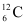and.
The electronic configuration of is2, 4.
The electronic configuration of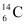is 2,4.
[Isotopes have the same electronic configuration]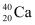and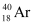are a pair of isobars
The electronic configuration ofis 2, 8, 8, 2.
The electronic configuration ofis 2, 8, 8.

Question 16. Compare the properties of electrons, protons and neutrons.

Solution :

Question 17. What are the limitations of J.J. Thomson’s model of the atom?
Solution : According to J.J. Thomson’s model of an atom, an atom consists of a positively charged sphere with electrons embedded in it. However, it was later found that the positively charged particles reside at the centre of the atom called the nucleus, and the electrons revolve around the nucleus.

Question 18. What are the limitations of Rutherford’s model of the atom?
Solution : According to Rutherford’s model of an atom, electrons revolve around the nucleus in fixed orbits. But, an electron revolving in circular orbits will not be stable because during revolution, it will experience acceleration. Due to acceleration, the electrons will lose energy in the form of radiation and fall into the nucleus. In such a case, the atom would be highly unstable and collapse.

Question 19. What are the limitations of Rutherford’s model of the atom?
Solution : Rutherford’s model of atom didn’t explain the stability of atom because as per his model revolving charged electrons while moving through orbit should emit energy and this energy loss will shrink the orbit and ultimately the electron would hit the nucleus and thus atom is unstable but it is not true.

Question 20. Describe Bohr’s model of the atom.
Solution : To remove the drawbacks of Rutherford’s atomic model and to explain structure of atom in detail Neils Bohr in 1912 proposed a model of atom. The special features of Bohr’s model are given below:
i) An electron revolves in the orbit of atom with well-defined energy.
ii) Energy of orbits increases from inner shell to the outer shells i.e. energy for orbit nearest the nucleus is lowest.
iii) If energy is supplied then electron moves from lower orbit ot the higher orbit and if an electron jumps from higher orbit (energy level) to the lower orbit (energy level) then energy is radiated as electromagnetic waves.
iv) Each orbit or shell represents an energy level. Such orbits are represented as K,L,M,N,O……….. and named from centre to outwards.
v) The shell or orbits are associated with certain amount of energy and energy of orbits/shells increases from inward to outwards.eg K<L<M<N<O…………

Question 21. Compare all the proposed models of an atom given in this chapter.
Solution :

Question 22. Summarize the rules for writing of distribution of electrons in various shells for the first eighteen elements.
Solution : The rules for writing of the distribution of electrons in various shells for the first eighteen elements are given below.
(i) The maximum number of electrons that a shell can accommodate is given by the formula ‘2n2’, where ‘n’ is the orbit number or energy level index (n = 1, 2, 3…).
The maximum number of electrons present in an orbit of n = 1 is given by 2n2 = 2×1= 2
Similarly, for second orbit, it is 2n2 = 2×22 = 8
For third orbit, it is 2n= 2×32 = 18
And so on……
(ii) The outermost orbit can be accommodated by a maximum number of 8 electrons.
(iii) Shells are filled with electrons in a stepwise manner i.e., the outer shell is not occupied with electrons unless the inner shells are completely filled with electrons.

Question 23. Define valency by taking examples of silicon and oxygen.
Solution : That number of electrons (present in outermost shell) /valence electrons which an atom gives or takes or shares to complete its octet, or acquire stable configuration. Therefore, valency of sulphur = 16= 2,8,6= 2 electrons can be gained easily hence valency is 2.
valency of oxygen = 8 = 2,6 = 2 electrons can be gained easily hence valency is 2.

Question 24. Explain with examples
(i) Atomic number,
(ii) Mass number,
(iii) Isotopes and
iv) Isobars.
Give any two uses of isotopes.

Solution :
i) Atomic number = It is the number of protons present inside nucleus of the atom.
It is represented as Z. For eg: for hydrogen Z= 1, because in hydrogen atom the number of protons is 1.
ii) Mass number = It is the total number of protons and neutrons present inside the nucleus of an atom and is represented by A = P + N mass of carbon is 12 u because it has 6 protons and 6 neutrons, 6 u + 6 u = 12 u.
iii) Isotopes = They are atoms of the same element and have same atomic number but different mass number/atomic mass. for example: carbon,
iv) Isobars = They are atoms of different elements having same mass number but different atomic number. for example, calcium, atomic number 20, and argon, atomic number 18. The number of electrons in these atoms is different, but the mass number of both these elements is 40. That is, the total number of neutrons is the same in the atoms of this pair of elements.
Two uses of isotopes are as follows:
(i) An isotope of uranium is used as a fuel in nuclear reactors.
(ii) An isotope of cobalt is used in the treatment of cancer.

Question 25. Nahas completely filled K and L shells. Explain.

Solution :
Na has atomic number 11, so its electronic configuration is = 2,8,1
When it gives away its outermost shell single electron it changes to Na+ =10= 2,8
The above configuration indicates completely filled K,L shells.

Question 26. If bromine atom is available in the form of, say, two isotopes(49.7%) and(50.3%), calculate the average atomic mass of bromine atom.

Solution :
It is given that two isotopes of bromine are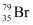(49.7%) and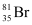(50.3%). Then, the average atomic mass of bromine atom is given by:

Question 27. The average atomic mass of a sample of an element X is 16.2 u. What are the percentages of isotopesin the sample?

Solution :
Since average atomic mass =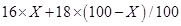16.2=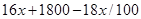1620 =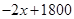2x= 1800 – 1620
x = 180/2 = 90
When 90% is the X-16 sample so for X-18 sample % = 100-90=10%

Question 28. If Z = 3, what would be the valency of the element? Also, name the element.

Solution :
When Z = 3, so that element has 3 electrons in its shells distributed as = 2, 1. Its valency is 1 because it can easily give away its outermost single electron and the element is Lithium(Li).

Question 29.
Composition of the nuclei of two atomic species X and Y are given as under
Give the mass numbers of X and Y. What is the relation between the two species?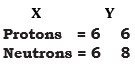Solution :
The mass number of X = 6+6 = 12
The mass number of Y = 6+8 = 14
since their number of protons are same but atomic mass are different so they are isotopes.

Question 30. For the following statements, write T for True and F for False.
(a) J.J. Thomson proposed that the nucleus of an atom contains only nucleons.
(b) A neutron is formed by an electron and a proton combining together. Therefore, it is neutral.
(c) The mass of an electron is about 12000 times that of proton.
(d) An isotope of iodine is used for making tincture iodine, which is used as a medicine.

Solution :
(a) false
(b) true
(c) true
(d) false

Question 31. Rutherford’s alpha-particle scattering experiment was responsible for the discovery of
(a) Atomic Nucleus
(b) Electron
(c) Proton
(d) Neutron

Solution : (c) proton

Question 32. Isotopes of an element have
(a) the same physical properties
(b) different chemical properties
(c) different number of neutrons
(d) different atomic numbers.

Solution : (a) the same physical properties

Question 33. Number of valence electrons in Cl– ion are :
(a) 16
(b) 8
(c) 17
(d) 18

Solution : (b) 8

Question 34. Which one of the following is a correct electronic configuration of sodium?
(a) 2,8
(b) 8,2,1
(c) 2,1,8
(d) 2,8,1.

Solution : (a) 2,8,1

Question 35. Complete the following table.

Solution :

## Conclusions for NCERT SOLUTIONS FOR CLASS 9 SCIENCE CHAPTER 4 STRUCTURE OF ATOM

SWC academic staff has developed NCERT answers for this chapter of the ninth grade science curriculum. We have solutions prepared for all the ncert questions of this chapter. The answers, broken down into steps, to all of the questions included in the NCERT textbook’s chapter are provided here. Read this chapter on theory. Be certain that you have read the theory section of this chapter of the NCERT textbook and that you have learnt the formulas for the chapter that you are studying.